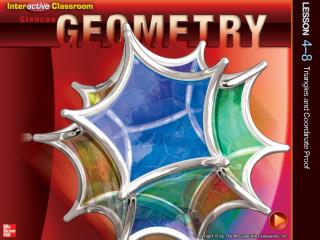DownloadDownload PresentationSplash Screen

# Splash Screen

Télécharger la présentation## Splash Screen

- - - - - - - - - - - - - - - - - - - - - - - - - - - E N D - - - - - - - - - - - - - - - - - - - - - - - - - - -
##### Presentation Transcript

1. Splash Screen

2. Five-Minute Check (over Lesson 4–7) CCSS Then/Now New Vocabulary Example 1: Position and Label a Triangle Key Concept: Placing Triangles on Coordinate Plane Example 2: Identify Missing Coordinates Example 3: Write a Coordinate Proof Example 4: Real-World Example: Classify Triangles Lesson Menu

3. Identify the type of congruence transformation shown as a reflection, translation, or rotation. A. reflection B. translation C. rotation 5-Minute Check 1

4. Identify the type of congruence transformation shown as a reflection, translation, or rotation. A. reflection B. translation C. rotation 5-Minute Check 2

5. Graph triangles LMN and PQR with vertices L(–4, 5), M(–4, 1), N(0, 3), and P(1, –1),Q(1, –5), and R(5, –3). Then identify the transformation. A. reflection B. translation C. rotation 5-Minute Check 3

6. Rectangle RSTU has vertices at (0, 0), (0, 4), (6, 4), and (6, 0). Which of the following is a vertex of the rectangle reflected over the x-axis? A. (–6, 0) B. (–4, –6) C. (0, –4) D. (–4, 0) 5-Minute Check 4

7. Content Standards G.CO.10 Prove theorems about triangles. G.GPE.4 Use coordinates to prove simple geometric theorems algebraically. Mathematical Practices 3 Construct viable arguments and critique the reasoning of others. 2 Reason abstractly and quantitatively. CCSS

8. You used coordinate geometry to prove triangle congruence. • Position and label triangles for use in coordinate proofs. • Write coordinate proofs. Then/Now

9. coordinate proof Vocabulary

10. Position and label right triangleXYZ with leg d units long on the coordinate plane. Position and Label a Triangle Use the origin as vertex X of the triangle. Place the base of the triangle along the positive x-axis. Position the triangle in the first quadrant. Since Z is on the x-axis, its y-coordinate is 0. Its x-coordinate is d because the base is d units long. Example 1

11. Position and Label a Triangle Since triangle XYZ is a right triangle, the x-coordinate of Y is 0. We cannot determine the y-coordinate, so call it b. Answer: Example 1

12. Which picture on the following slide would be the best way to position and label equilateral triangle ABC with side w units long on the coordinate plane? Example 1

13. A. B. C. D. Example 1

14. Concept

15. The y-coordinate for S is the distance from R to S. Since ΔQRS is an isosceles right triangle, Identify Missing Coordinates Name the missing coordinates of isosceles right triangle QRS. Q is on the origin, so its coordinates are (0, 0). The x-coordinate of S is the same as the x-coordinate for R, (c, ?). The distance from Q to R is c units. The distance from R to S must be the same. So, the coordinates of S are (c, c). Answer:Q(0, 0); S(c, c) Example 2

16. Name the missing coordinates of isosceles right ΔABC. A.A(d, 0); C(0, 0) B.A(0, f); C(0, 0) C.A(0, d); C(0, 0) D.A(0, 0); C(0, d) Example 2

17. Given:ΔXYZ is isosceles. Write a Coordinate Proof Write a coordinate proof to prove that the segment that joins the vertex angle of an isosceles triangle to the midpoint of its base is perpendicular to the base. The first step is to position and label an isosceles triangle on the coordinate plane. Place the base of the isosceles triangle along the x-axis. Draw a line segment from the vertex of the triangle to its base. Label the origin and label the coordinates, using multiples of 2 since the Midpoint Formula takes half the sum of the coordinates. Prove: Example 3

18. Proof:By the Midpoint Formula, the coordinates of W, the midpoint of , is The slope of or undefined. The slope of is therefore, . Write a Coordinate Proof Example 3

19. Finish the following coordinate proof to prove that the segment drawn from the right angle to the midpoint of the hypotenuse of an isosceles right triangle is perpendicular to the hypotenuse. Example 3

20. Proof:The coordinates of the midpoint D are The slope of is or 1. The slope of or –1, therefore because ____. ? A. their slopes are opposite. B. the sum of their slopes is zero. C. the product of their slopes is –1. D. the difference of their slopes is 2. Example 3

21. Classify Triangles DRAFTINGWrite a coordinate proof to prove that the outside of this drafter’s tool is shaped like a right triangle. The length of one side is 10 inches and the length of another side is 5.75 inches. Example 4

22. Proof:The slope of or undefined. The slope of or 0, therefore ΔDEF is a right triangle. The drafter’s tool is shaped like aright triangle. Classify Triangles Example 4

23. FLAGS Tracy wants to write a coordinate proof to prove this flag is shaped like an isosceles triangle. The altitude is 16 inches and the base is 10 inches. Example 4

24. What ordered pair should she use for point C? A. (10, 10) B. (10, 5) C. (16, 10) D. (16, 5) Example 4

25. End of the Lesson